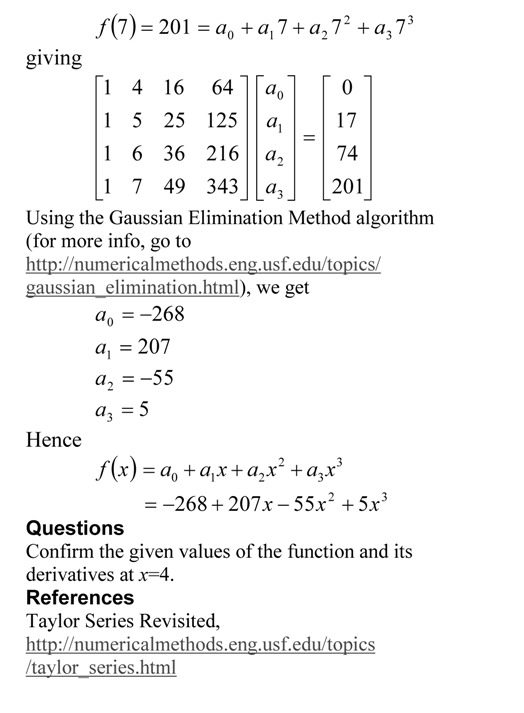## How do I do that in MATLAB

HOW DO I DO THAT IN MATLAB SERIES?

## Using Taylor polynomial to approximately solve an ordinary differential equation

Taylor polynomial is an essential concept in understanding numerical methods. Examples abound and include finding accuracy of divided difference approximation of derivatives and forming the basis for Romberg method of numerical integration.

In this example, we are given an ordinary differential equation and we use the Taylor polynomial to approximately solve the ODE for the value of the dependent variable at a particular value of the independent variable. As a homework assignment, do the following.
1) compare the approximate solution with the exact one, and
2) get another approximate solution by using a third order Taylor polynomial.

You can visit the above example by opening a pdf or video file.

This post is brought to you by Holistic Numerical Methods: Numerical Methods for the STEM undergraduate at http://nm.MathForCollege.com, the textbook on Numerical Methods with Applications available from the lulu storefront, the textbook on Introduction to Programming Concepts Using MATLAB, and the YouTube video lectures available at http://nm.MathForCollege.com/videos.  Subscribe to the blog via a reader or email to stay updated with this blog. Let the information follow you.

## Taylor Series in Layman’s terms

The Taylor series for a function f(x) of one variable x is given by$f(x+h) = f(x) + f '(x)h +f ''(x) h^2/2!+ f ''' (x)h^3/3! + .............$

What does this mean in plain English?
As Archimedes would have said (without the fine print), “Give me the value of the function at a single point, and the value of all (first, second, and so on) its derivatives, and I can give you the value of the function at any other point”.
It is very important to note that the Taylor series is not asking for the expression of the function and its derivatives, just the value of the function and its derivatives at a single point.

Now the fine print: Yes, all the derivatives have to exist and be continuous between x (the point where you are) to the point, x+h where you are wanting to calculate the function at. However, if you want to calculate the function approximately by using the$n^{th}$ order Taylor polynomial, then$1^{st}$,$2^{nd}$ …….,$n^{th}$ derivatives need to exist and be continuous in the closed interval [x, x+h] , while the$n+1^{th}$ derivative needs to exist and be continuous in the open interval (x, x+h).

Reference: Taylor Series Revisited

This post is brought to you by

## Taylor Series Exercise – Method 3

Taylor series is an important concept for learning numerical methods – not only for understanding how trigonometric and transcendental functions are calculated by a computer, but also for error analysis in numerical methods. I asked the question below in the first test in the course, and half of the students did not get to the final answer. In a previous blog, I showed you the method that most instructors would use. See how some students approached (another approach) the problem.The pdf file of the solution is also available.

This post is brought to you by

## Taylor Series Exercise – Method 2

Taylor series is an important concept for learning numerical methods – not only for understanding how trigonometric and transcendental functions are calculated by a computer, but also for error analysis in numerical methods. I asked the question below in the first test in the course, and half of the students did not get to the final answer. In a previous blog, I showed you the method that most instructors would use. See how some students approached the problem.The pdf file of the solution is also available.

This post is brought to you by

## Taylor Series Exercise – Method 1

Taylor series is an important concept for learning numerical methods – not only for understanding how trigonometric and transcendental functions are calculated by a computer, but also for error analysis in numerical methods.  I asked the question below in the first test in the course, and half of the students did not get to the final answer.The pdf file of the solution is also available.

This post is brought to you by

## Accuracy of Taylor series

So how many terms should I use in getting a certain pre-determined accuracy in a Taylor series. One way is to use the formula for the Taylor’s theorem remainder and its bounds to calculate the number of terms. This is shown in the example below.This post is brought to you by Holistic Numerical Methods: Numerical Methods for the STEM undergraduate at http://numericalmethods.eng.usf.edu.

An abridged (for low cost) book on Numerical Methods with Applications will be in print (includes problem sets, TOC, index) on December 10, 2008 and available at lulu storefront.

## Taylor Series Revisited

Taylor series is a very important concept that is used in numerical methods. From the concept of truncation error to finding the true error in Trapezoidal rule, having a clear understanding of Taylor series is extremely important. Other places in numerical methods where Taylor series concept is used include: the derivation of finite difference formulas for derivatives, finite difference method of solving differential equations, error in Newton Raphson method of solving nonlinear equations, Newton divided difference polynomial for interpolation, etc.

I have written a short chapter on Taylor series. After reading the chapter, you should be able to:

1. understand the basics of Taylor’s theorem,

2. see how transcendental and trigonometric functions can be written as Taylor’s polynomial,

3. use Taylor’s theorem to find the values of a function at any point, given the values of the function and all its derivatives at a particular point,

4. errors and error bounds of approximating a function by Taylor series,

5. revisit the chapter whenever Taylor’s theorem is used to derive or explain numerical methods for various mathematical procedures.

This post is brought to you by Holistic Numerical Methods: Numerical Methods for the STEM undergraduate at http://numericalmethods.eng.usf.edu.

An abridged (for low cost) book on Numerical Methods with Applications will be in print (includes problem sets, TOC, index) on December 10, 2008 and available at lulu storefront.